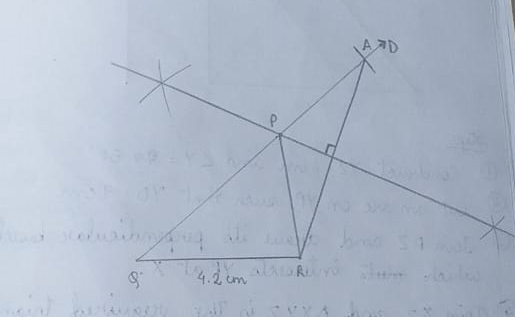# Maharashtra Board Class 9 Math Part 2 Chapter 4 Quadrilaterals Solution

## Maharashtra Board Class 9 Math Part 2 Solution Chapter 4 – Quadrilaterals

Balbharati Maharashtra Board Class 9 Math Part 2 Solution Chapter 4: Quadrilaterals. Marathi or English Medium Students of Class 9 get here Quadrilaterals full Exercise Solution.

 Std Maharashtra Class 9 Subject Math Part 2 Solution Chapter Quadrilaterals

### Practice Set 4.1

(1) Construct PQR, in which QR = 4.2 cm, mQ = 40° and PQ + PR = 8.5 cmSteps –

(1) Construct QR = 4.2 cm and ∠Q = 40°

(2) Cut an are QA on QD such that AQ = 8.5 cm

(3) Join AR and draw perpendicular bisector that must AQ at P

(4) Joining PR we get the △PQR

(2) Construct XYZ, in which YZ = 6 cm, XY + XZ = 9 cm. XYZ = 50°Steps –

(1) Construct YZ = 6cm and ∠Y = 50°

(2) Cut an arc on YP such that YD = 9cm

(3) Join DZ and draw its perpendicular bisector which intersects YP at x.

(4) Join XZ and △XYZ is the required triangle

(3) Construct ABC, in which BC = 6.2 cm, ACB = 50°, AB + AC = 9.8 cmSteps –

(1) Construct BC = 6.2 cm and ∠C = 50°

(2) Cut an are on CP such that CD = 9.8 cm

(3 Join BD and draw its perpendicular bisector which intersects CD at A

(4) Joining AB we get △ABC

(4) Construct ABC, in which BC = 3.2 cm, ACB = 45° and perimeter of ABC is 10 cm

Solution:

Perimeter of △ABC = 10cm

= AB + BC + AC = 10cm

= AB + AC = 10 – 3.2

= 6.8 cm

Steps –

(1) Construct BC = 3.2 cm and ∠C = 45°

(2) Cut an arc of CP such that CD = 6.8 cm

(3) Join BD and draw its perpendicular bisector which intersects CD at A

(4) Joining AB, we get △ABC### Practice Set 4.2

(1)  Construct XYZ, such that YZ = 7.4 cm, XYZ = 45° and XY – XZ = 2.7 cm.

Solution:Steps –

(1) Construct YZ = 7.4 cm and ∠Y = 45°

(2) Cut are on YP such that YD = 3 cm

(3) Join DZ and draw it’s perpendicular bisector which intersects YP at X

(4) Joining XZ we get the required △XYZ

(2) Construct PQR, such that QR = 6.5 cm, PQR = 40° and PQ – PR = 2.5 cm.

Solution:Steps –

(1) Construct QR = 6.5 cm and ∠Q = 40°

(2) Cut arc on QM such that QD = 2.5 cm

(3) Join DR and draw it’s perpendicular bisector which intersects QM at P

(4) Joining PR we get △PQR

(3) Construct ABC, such that BC = 6 cm, ABC = 100° and AC – AB = 2.5 cm.

Solution:Steps –

(1) Construct BC = 6 cm & ∠B = 100°

(2) Cut arc on BS such that BD = 2.5 cm

(3) Join CD and draw it’s perpendicular bisector which intersects BP at A

(4) Join AC we get △ABC

### Practice Set 4.3

(1) Construct PQR, in which Q = 70°, R = 80° and PQ + QR + PR = 9.5 cm.

Solution:

Given, ∠Q = 70°

∴ ∠PAQ = ½ CQ = 35° and ∠R = 80°

∠PBR = ½ ∠R = 40°Steps –

(1) Construct AB = 9.5 cm and ∠A = 35° and ∠B = 40°

(2) Let, the line from A and B meet at P

(3) Draw perpendicular bisectors of AP and PB which intersects AB at Q and R respectively

(4) Join PQ and QR, we get △PQR

(2) Construct XYZ, in which Y = 58°, X = 46° and perimeter of triangle is 10.5 cm.

Solution:

Given, ∠Y = 58°, ∴ ∠A = 58°/2 = 29°

∠X = 46° ∴ ∠B = 46°/2 = 23°Steps –

(1) Construct AB = 10.5 cm and ∠A = 29° & ∠B = 23°

(2) Let, lines from A & B meet at

(3) Draw perpendicular bisectors of AZ and BZ. Which intersects A at Y and X respectively

(4) Join XZ and YZ we, get △XYZ

(3) Construct LMN, in which M = 60°, N = 80° and LM + MN + NL = 11 cm.

Solution:

Given, ∠M = 60°, ∠A = 30°

∠N = 80°, ∠B = 40°Steps –

(1) Construct AB = 11cm and ∠A = 30° & ∠B = 40°

(2) Let, lines from A & B meet at L

(3) Draw perpendicular bisectors of AL and BL which intersects AB at M and N respectively

(4) Join LM and LN, we get △LMN

Updated: September 22, 2021 — 9:52 pm